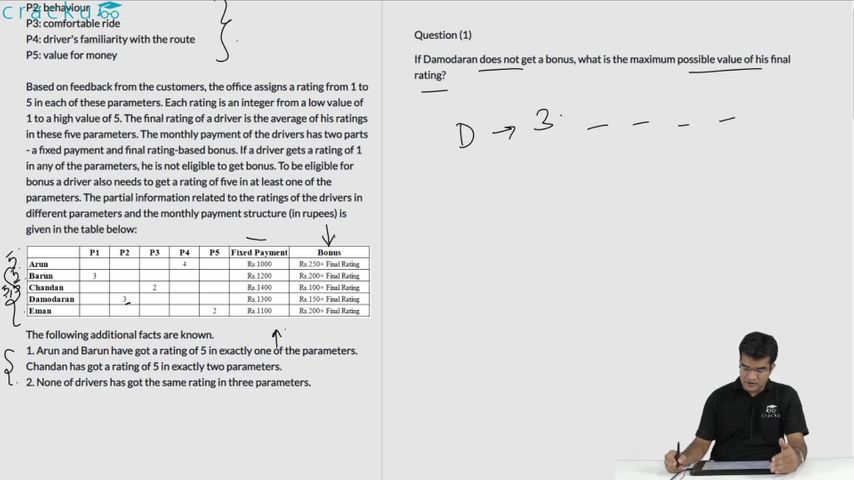### CAT 2020 Question Paper (Slot 1) Question 37

Instructions

The local office of the APP-CAB company evaluates the performance of five cab drivers, Arun, Barun, Chandan, Damodaran, and Eman for their monthly payment based on ratings in five different parameters (P1 to P5) as given below:
P1: timely arrival
P2: behaviour
P3: comfortable ride
P4: driver's familiarity with the route
P5: value for money

Based on feedback from the customers, the office assigns a rating from 1 to 5 in each of these parameters. Each rating is an integer from a low value of 1 to a high value of 5. The final rating of a driver is the average of his ratings in these five parameters. The monthly payment of the drivers has two parts - a fixed payment and final rating-based bonus. If a driver gets a rating of 1 in any of the parameters, he is not eligible to get bonus. To be eligible for bonus a driver also needs to get a rating of five in at least one of the parameters. The partial information related to the ratings of the drivers in different parameters and the monthly payment structure (in rupees) is given in the table below:

The following additional facts are known.
1. Arun and Barun have got a rating of 5 in exactly one of the parameters. Chandan has got a rating of 5 in exactly two parameters.
2. None of drivers has got the same rating in three parameters.

Question 37

# If Damodaran does not get a bonus, what is the maximum possible value of his final rating?

Solution

Based on the given conditions, Damodaran misses out on the bonus if he gets a rating of 1 in any of the five parameters. He additionally needs to obtain a rating of 5 in at least one of the parameters. Thus, the maximum value of the range of ratings that he can acquire would be 1+3(given)+5+5+5. However, based on condition 2, he can have similar ratings in only two of the parameters. Thus, the maximum value of the final rating would be (1+3+5+5+4) /5 = 18/5 = 3.6. Hence, Option C is the correct answer.

### View Video Solution• All Quant CAT Formulas and shortcuts PDF
• 30+ CAT previous papers with solutions PDF

##### Pranshu Soni

1 week, 4 days ago

He can also get P1=5, P2=3(given), P3=5, P4=1, P5=5, thus total rating would be (5+3+5+1+5)/5 = 19/5 = 3.8

then option D would be correct.